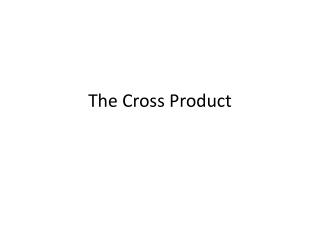DownloadDownload PresentationThe Cross Product

# The Cross Product

Download Presentation## The Cross Product

- - - - - - - - - - - - - - - - - - - - - - - - - - - E N D - - - - - - - - - - - - - - - - - - - - - - - - - - -
##### Presentation Transcript

1. The Cross Product

2. 3. Find (a) v x w, (b) w x v, (c) w x w, and (d) v x v(Similar to p.370 #15-22)

3. 4. Find (a) v x w, (b) w x v, (c) w x w, and (d) v x v(Similar to p.370 #15-22)

4. 5. Use the given vectors u, v, and w to find each expression.u = 3i – j + 2kv = 4i + j – 5kw = i + j + k(Similar to p.370 #23-40)

5. 6. Use the given vectors u, v, and w to find each expression.u = 3i – j + 2kv = 4i + j – 5kw = i + j + k(Similar to p.370 #23-40)

6. 7. Use the given vectors u, v, and w to find each expression.u = 3i – j + 2kv = 4i + j – 5kw = i + j + k(Similar to p.370 #23-40)

7. 8. Use the given vectors u, v, and w to find each expression.u = 3i – j + 2kv = 4i + j – 5kw = i + j + k(Similar to p.370 #23-40)

8. 9. Use the given vectors u, v, and w to find each expression.u = 3i – j + 2kv = 4i + j – 5kw = i + j + k(Similar to p.370 #41-44)vector orthogonal to both u and v (Hint u x v is orthogonal to both)

9. 11. Find the area of the parallelogram with vertices P1, P2, P3, and P4(Similar to p.370 #49-52)